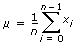Mean Method

Measurement Studio 2012 for Visual Studio 2010 Help

Edition Date: August 2012

Part Number: 372636F-01

»View Product InfoDownload Help (Windows Only)

Computes the mean of the values in the input array.

Namespace: NationalInstruments.Analysis.Math

Assembly: NationalInstruments.Analysis.Enterprise (in NationalInstruments.Analysis.Enterprise.dll) Version: 12.0.40.318

Syntax

Visual Basic (Declaration)
<SecurityPermissionAttribute(SecurityAction.Demand, Flags := SecurityPermissionFlag.UnmanagedCode)> _
Public Shared Function Mean ( _
inputData As Double() _
) As Double

C#
[SecurityPermissionAttribute(SecurityAction.Demand, Flags = SecurityPermissionFlag.UnmanagedCode)]
public static double Mean(
double[] inputData
)

Parameters

inputData
Type: System.Double []
The input array.

Return Value

The average of the values in inputData.

Exceptions

ExceptionCondition
ArgumentException The number of samples in inputData is equal to zero.
ArgumentNullException inputData is null (Nothing in Visual Basic).
DllNotFoundException The analysis library cannot be found.
EntryPointNotFoundException A required operation in the analysis library cannot be found.

Remarks

This method uses the following formula:where n is the number of elements in X, the inputData parameter.

Examples

The following example calculates the mean of the generated noise signal.
VB.NET
Dim inputData(1999) As Double
Dim mean As Double
Dim gaussianNoise As GaussianNoiseSignal = New GaussianNoiseSignal(1.0, 17)

' Generate a Gaussian noise signal that represents inputData
inputData = gaussianNoise.Generate(1000.0, 2000)

mean = Statistics.Mean(inputData)
C#
double[] inputData = new double;
double mean;
GaussianNoiseSignal gaussianNoise = new GaussianNoiseSignal(1.0, 17);

// Generate a Gaussian noise signal that represents inputData
inputData = gaussianNoise.Generate(1000.0, 2000);

mean = Statistics.Mean(inputData);